# NES Essential Academic Skills Math: Triangles Chapter Exam

Exam Instructions:

Choose your answers to the questions and click 'Next' to see the next set of questions. You can skip questions if you would like and come back to them later with the yellow "Go To First Skipped Question" button. When you have completed the practice exam, a green submit button will appear. Click it to see your results. Good luck!

Answered 0 of 30

### Page 1

#### Question 1 1. What type of line segment is represented by the vertical dotted line in the pictured triangle?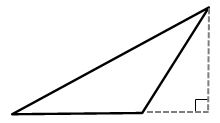#### Question 2 2. If angle a is 66 degrees and angle b is 54 degrees, what is the measure of angle d?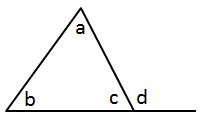#### Question 3 3. Determine if triangle VEZ is similar to triangle MAG.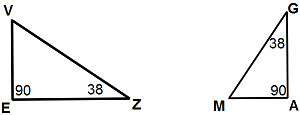#### Question 5 5. Is triangle ABC similar to triangle DEF?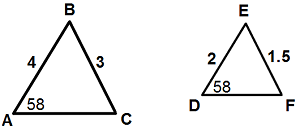### Page 2

#### Question 6 6. If angle a is 80 degrees, what is the measure of angle d?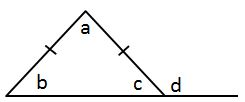#### Question 8 8. In the pictured triangle, which of the following lines is an altitude?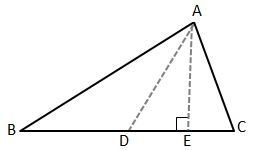#### Question 9 9. Josh claims that these triangles are similar by SSS. Do you agree or disagree with Josh?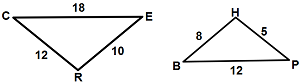#### Question 10 10. Identify x and y in the pictured triangle.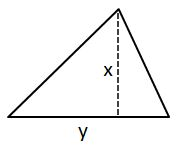### Page 3

#### Question 11 11. In the pictured triangle, what is side B, relative to angle x?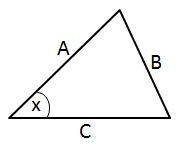#### Question 12 12. What type of triangle is pictured?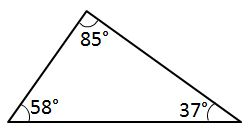#### Question 13 13. If angle a is 60 degrees, what is the measure of angle b?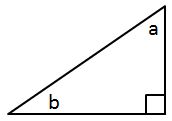#### Question 14 14. What type of line segment is represented by the dotted line in the pictured triangle?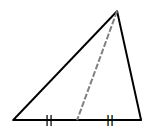### Page 4

#### Question 16 16. What type of triangle is pictured?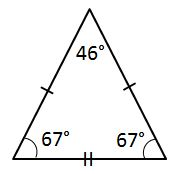#### Question 17 17. What type of line segment is represented by the dotted line in the pictured triangle?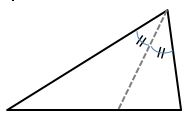#### Question 18 18. Mary claims that the triangles below are similar by AA. Do you agree or disagree with Mary?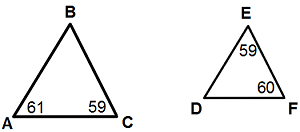#### Question 19 19. What type of triangle is pictured?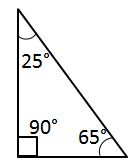### Page 5

#### Question 21 21. If angle a is 30 degrees and angle d is 70 degrees, what is the measure of angle b?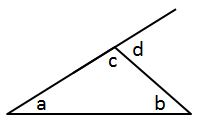#### Question 24 24. If angle a is 68 degrees and angle b is 37 degrees, what is the measure of angle c?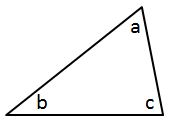#### Question 25 25. In the pictured triangle, what is side A, relative to angle x?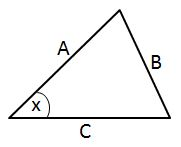### Page 6

#### Question 26 26. What type of triangle is pictured?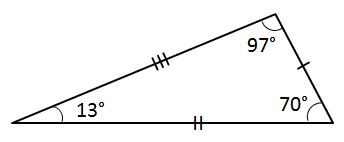#### Question 28 28. Conclude whether triangle BEN is similar to triangle MAT.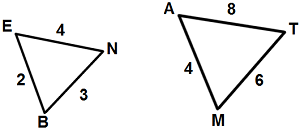#### Question 29 29. What type of triangle is pictured?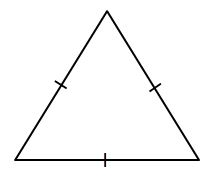#### Question 30 30. In the pictured triangle, identify the altitude(s).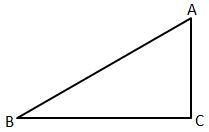#### NES Essential Academic Skills Math: Triangles Chapter Exam Instructions

Choose your answers to the questions and click 'Next' to see the next set of questions. You can skip questions if you would like and come back to them later with the yellow "Go To First Skipped Question" button. When you have completed the practice exam, a green submit button will appear. Click it to see your results. Good luck!

Support